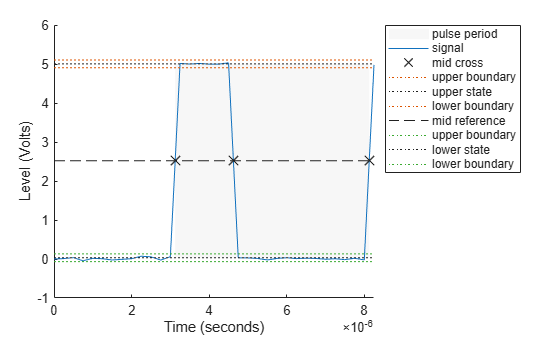Documentation

# pulseperiod

Period of bilevel pulse

## Syntax

```P = pulseperiod(X) P = pulseperiod(X,FS) P = pulseperiod(X,T) [P,INITCROSS] = pulseperiod(...) [P,INITCROSS,FINALCROSS] = pulseperiod(...) [P,INITCROSS,FINALCROSS,NEXTCROSS] = pulseperiod(...) [P,INITCROSS,FINALCROSS,NEXTCROSS,MIDLEV] = pulseperiod(...) [P,INITCROSS,FINALCROSS,NEXTCROSS,MIDLEV] = pulseperiod(...,Name,Value) pulseperiod(...) ```

## Description

`P = pulseperiod(X)` returns a vector, `P`, containing the difference between the mid-reference level instants of the initial transition of each positive-polarity pulse and the next positive-going transition in the bilevel waveform, `X`. If `pulseperiod` does not find two positive-polarity transitions, `P` is empty. To determine the transitions for each pulse, `pulseperiod` estimates the state levels of the input waveform by a histogram method and identifies all regions which cross the upper-state boundary of the low state and the lower-state boundary of the high state. The low-state and high-state boundaries are expressed as the state level plus or minus a multiple of the difference between the state levels. See State-Level Tolerances. Because `pulseperiod` uses interpolation to determine the mid-reference level instants, `P` may contain values that do not correspond to sampling instants of the bilevel waveform, `X`.

`P = pulseperiod(X,FS)` specifies the sample rate in hertz as a positive scalar. The first sample instant in `X` corresponds to t=0. Because `pulseperiod` uses interpolation to determine the mid-reference level instants, `P` may contain values that do not correspond to sampling instants of the bilevel waveform, `X`.

`P = pulseperiod(X,T)` specifies the sampling instants in a vector equal in length to `X`. Because `pulseperiod` uses interpolation to determine the mid-reference level instants, `P` may contain values that do not correspond to sampling instants of the bilevel waveform, `X`.

```[P,INITCROSS] = pulseperiod(...)``` returns the mid-reference level instants of the first transition of each pulse.

```[P,INITCROSS,FINALCROSS] = pulseperiod(...)``` returns the mid-reference level instants of the final transition of each pulse.

```[P,INITCROSS,FINALCROSS,NEXTCROSS] = pulseperiod(...)``` returns the mid-reference level instants of next detected transition after each pulse.

```[P,INITCROSS,FINALCROSS,NEXTCROSS,MIDLEV] = pulseperiod(...)``` returns the mid-reference level,`MIDLEV`.

```[P,INITCROSS,FINALCROSS,NEXTCROSS,MIDLEV] = pulseperiod(...,Name,Value)``` returns the pulse periods with additional options specified by one or more `Name,Value` pair arguments.

`pulseperiod(...)` plots the signal and darkens every other identified pulse. It marks the location of the mid crossings, and their associated reference level. The state levels and their associated lower and upper boundaries (adjustable by the `Name,Value` pair with name `'Tolerance'`) are also plotted.

## Input Arguments

 `X` Bilevel waveform. If the waveform, `X`, does not contain at least two transitions, `pulseperiod` outputs an empty matrix. `FS` Sample rate in hertz. `T` Vector of sample instants. The length of `T` must equal the length of the bilevel waveform, `X`.

### Name-Value Pair Arguments

 `'MidPercentReferenceLevel'` Mid-reference level as a percentage of the waveform amplitude. Default: 50 `'Polarity'` Pulse polarity. Specify the polarity as `'positive'` or `'negative'`. If you specify `'positive'`, `pulseperiod` looks for pulses whose initial transition is positive-going (positive polarity). If you specify `'negative'`, `pulseperiod` looks for pulses whose initial transition is negative-going (negative polarity). Default: `'positive'` `'StateLevels'` Low- and high-state levels. `StateLevels` is a 1-by-2 real-valued vector. The first element is the low-state level. The second element is the high-state level. If you do not specify low and high-state levels, `pulseperiod` estimates the state levels from the input waveform using the histogram method. `'Tolerance'` Tolerance levels (lower and upper state boundaries) expressed as a percentage. See State-Level Tolerances. Default: 2

## Output Arguments

 `P` Pulse period in seconds. The pulse period is defined as the time between the mid-reference level instants of two consecutive transitions. `INITCROSS` Mid-reference level instant of initial transition. `FINALCROSS` Mid-reference level instant of final transition. `NEXTCROSS` Mid-reference level instant of the first pulse transition after the final transition of the preceding pulse. `MIDLEV` Waveform value that corresponds to the mid-reference level.

## Examples

collapse all

Compute the pulse period of a bilevel waveform with two positive-polarity transitions. The sample rate is 4 MHz.

```load('pulseex.mat','x','t') p = pulseperiod(x,t)```
```p = 5.0030e-06 ```

Annotate the pulse period on a plot of the waveform.

`pulseperiod(x,t);`Determine the mid-reference level instants that define the pulse period for a bilevel waveform.

```load('pulseex.mat','x','t') [~,initcross,~,nextcross] = pulseperiod(x,t)```
```initcross = 3.1240e-06 ```
```nextcross = 8.1270e-06 ```

Output the pulse period. Mark the mid-reference level instants on a plot of the data.

`pulseperiod(x,t)````ans = 5.0030e-06 ```

collapse all

### Mid-Reference Level

The mid-reference level in a bilevel waveform with low-state level, S1, and high- state level, S2, is

`${S}_{1}+\frac{1}{2}\left({S}_{2}-{S}_{1}\right)$`

### Mid-Reference Level Instant

Let y50% denote the mid-reference level.

Let t50%- and t50%+ denote the two consecutive sampling instants corresponding to the waveform values nearest in value to y50%.

Let y50%- and y50%+ denote the waveform values at t50%- and t50%+.

The mid-reference level instant is

`${t}_{50%}={t}_{50%}+\left(\frac{{t}_{50{%}_{+}}-{t}_{50{%}_{-}}}{{y}_{50{%}_{+}}-{y}_{50{%}_{-}}}\right)\left({y}_{50{%}_{+}}-{y}_{50{%}_{-}}\right)$`

### Pulse Polarity

If the initial transition of a pulse is positive-going, the pulse has positive polarity. The following figure shows a positive-polarity pulse.Equivalently, a positive-polarity (positive-going) pulse has a terminating state more positive than the originating state.

If the initial transition of a pulse is negative-going, the pulse has negative polarity. The following figure shows a negative-polarity pulse.Equivalently, a negative-polarity (negative-going) pulse has a originating state more positive than the terminating state.

### State-Level Tolerances

Each state level can have associated lower- and upper-state boundaries. These state boundaries are defined as the state level plus or minus a scalar multiple of the difference between the high state and the low state. To provide a useful tolerance region, the scalar is typically a small number such as 2/100 or 3/100. In general, theregion for the low state is defined as

``

whereis the low-state level andis the high-state level. Replace the first term in the equation withto obtain thetolerance region for the high state.

The following figure illustrates lower and upper 2% state boundaries (tolerance regions) for a positive-polarity bilevel waveform. The red dashed lines indicate the estimated state levels.## References

 IEEE® Standard on Transitions, Pulses, and Related Waveforms, IEEE Standard 181, 2003.